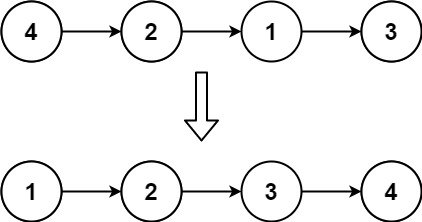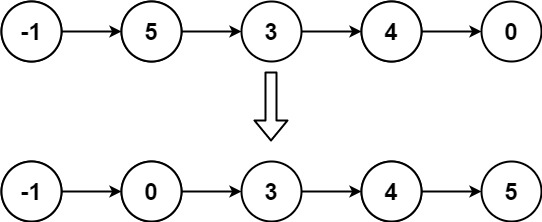## Algorithm

Problem Name: 147. Insertion Sort List

Given the `head` of a singly linked list, sort the list using insertion sort, and return the sorted list's head.

The steps of the insertion sort algorithm:

1. Insertion sort iterates, consuming one input element each repetition and growing a sorted output list.
2. At each iteration, insertion sort removes one element from the input data, finds the location it belongs within the sorted list and inserts it there.
3. It repeats until no input elements remain.

The following is a graphical example of the insertion sort algorithm. The partially sorted list (black) initially contains only the first element in the list. One element (red) is removed from the input data and inserted in-place into the sorted list with each iteration.Example 1:```Input: head = [4,2,1,3]
Output: [1,2,3,4]
```

Example 2:```Input: head = [-1,5,3,4,0]
Output: [-1,0,3,4,5]
```

Constraints:

• The number of nodes in the list is in the range `[1, 5000]`.
• `-5000 <= Node.val <= 5000`

## Code Examples

### #1 Code Example with C Programming

```Code - C Programming```

``````
#include <stdio.h>
#include <stdlib.h>
#include <time.h>

struct ListNode {
int val;
struct ListNode *next;
};

struct ListNode* insertionSortList(struct ListNode* head) {
if (head == NULL) return NULL;

struct ListNode *dummy = (struct ListNode *)malloc(sizeof(struct ListNode));
dummy->val = 0;
dummy->next = NULL;
struct ListNode *t = dummy;

struct ListNode *p;
struct ListNode *min_ptr, *min_prev; /* prev node of min node */
int min_val;

while (p) {
min_val = p->val;
min_ptr = p;
min_prev = NULL;

struct ListNode *q = p->next;
struct ListNode *prev = p;
while (q) {
if (q->val < min_val) {
min_val = q->val;
min_prev = prev;
min_ptr = q;
}
prev = q;
q = q->next;
}

if (p == min_ptr) { /* if min node is p, we don't need to modify list */
p = p->next;    /* just move forward p, and the min_prev is still */
}                   /* NULL now. */
else {
min_prev->next = min_ptr->next; /* take node from list */
}
t->next = min_ptr;
t = t->next;
}
t->next = NULL;

t = dummy->next;
free(dummy);

return t;
}
``````
Copy The Code &

Input

cmd

Output

cmd
[1,2,3,4]

### #2 Code Example with Java Programming

```Code - Java Programming```

``````
class Solution {
ListNode dummy = new ListNode(0);
ListNode prev = dummy;
ListNode next = null;
while (curr != null) {
next = curr.next;
while (prev.next != null && prev.next.val < curr.val) {
prev = prev.next;
}
curr.next = prev.next;
prev.next = curr;
prev = dummy;
curr = next;
}
return dummy.next;
}
}
``````
Copy The Code &

Input

cmd

Output

cmd
[1,2,3,4]

### #3 Code Example with Javascript Programming

```Code - Javascript Programming```

``````
const dummy = new ListNode()
let insert = dummy
while (cur && cur.next) {
if (cur.val < cur.next.val) {
cur = cur.next
continue
}
insert = dummy
while (insert.next.val < cur.next.val) {
insert = insert.next
}
const temp = cur.next
cur.next = temp.next
temp.next = insert.next
insert.next = temp
}
return dummy.next
}
``````
Copy The Code &

Input

cmd

Output

cmd
[-1,0,3,4,5]

### #4 Code Example with Python Programming

```Code - Python Programming```

``````
class Solution:
"""
:rtype: ListNode
"""
ref, ref.next, n = ListNode(-float("inf")), head, 0
inserted, curr, prev, n = ListNode(head.val), ref.next, ref, n+1
for i in range(n-1):
if inserted.val= n-2: curr.next = None
break
else:
prev, curr = curr, curr.next
if i == n-2: prev.next = inserted
return ref.next
``````
Copy The Code &

Input

cmd